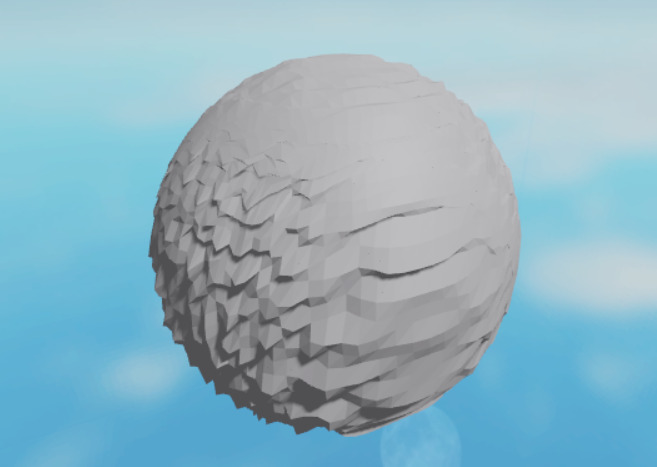# How to use perlin noise on a sphere?

So I’ve got some code that generates a giant sphere out of triangles. What I’m tryna do is make a sort of terrain on said sphere. I’ve got some basic terrain gen working, but it doesn’t take into account some sides. Here’s what a softer version of it looks like:

And if you can’t see the problem with that image, let me scale it up:As you can see, there’s a strip of terrain down the middle that doesn’t generate like the rest. (As well as the outsides pointing outwards, instead of upwards, relative to their starting position)
Here’s the code I use to find the perlin noise of any point:

``````pos1 = pos1+Vector3.new(math.noise(pos1.X/terrainDensity,pos1.Y/terrainDensity,seed)*terrainScale,math.noise(pos1.X/terrainDensity,pos1.Y/terrainDensity,seed)*terrainScale,math.noise(pos1.X/terrainDensity,pos1.Y/terrainDensity,seed)*terrainScale)
-- all three arguments for the `Vector3.new()` are the same
``````

And this is how it’s generated for each point. I’m assuming it has something to do with me only calculating the x and y value, therefore imposing a 2d noise structure onto the 3d sphere. But I can’t figure out how to make the noise generate properly for the 3d sphere structure. Any help would be appreciated!

1 Like

Fix was relatively simple, just created a map that combined all variations of the 2d maps, and it worked out nicely!

``````function perlinNoise(x,y,z)
local ab = math.noise(x/terrainDensity,y/terrainDensity,seed)
local bc = math.noise(y/terrainDensity,z/terrainDensity,seed)
local ac = math.noise(x/terrainDensity,z/terrainDensity,seed)

local abc = Vector3.new(ab,bc,ac)

local ba = math.noise(y/terrainDensity,x/terrainDensity,seed)
local cb = math.noise(z/terrainDensity,y/terrainDensity,seed)
local ca = math.noise(z/terrainDensity,x/terrainDensity,seed)

local cba = Vector3.new(ba,cb,ca)

local abcVector = abc+cba

return (abcVector/6)*terrainScale
end
``````1 Like